# ski-in / ski-out - Page 26

Viewing mode
750 results
• Slopes access :
• 50 -300m to the slopes
• Rooms :
• 4 rooms
• Total surface (sq.m) :
• 50 sq.m
•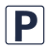•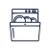•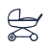•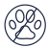• Slopes access :
• 50 -300m to the slopes
• Rooms :
• 4 rooms
•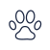•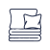••• Slopes access :
• 50 -300m to the slopes
• Rooms :
• Studio
• Studio + cabin(s)
• Total surface (sq.m) :
• 42 sq.m
•••• Slopes access :
• 50 -300m to the slopes
• Rooms :
• 3 rooms
• Total surface (sq.m) :
• 80 sq.m
•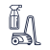••••• Slopes access :
• 50 -300m to the slopes
• Rooms :
• Studio
• Studio + cabin(s)
• Total surface (sq.m) :
• 24 sq.m
••••• Slopes access :
• 50 -300m to the slopes
• Slopes access :
• ski-in (-50 m)
• Rooms :
• Studio
• Total surface (sq.m) :
• 35 sq.m
•••• Slopes access :
• 50 -300m to the slopes
• Rooms :
• 2 rooms
• Total surface (sq.m) :
• 40 sq.m
•••• Slopes access :
• ski-in (-50 m)
• Rooms :
• 3 rooms
• 3 rooms + cabin(s)
• Total surface (sq.m) :
• 71 sq.m
••••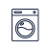••• Slopes access :
• ski-in (-50 m)
• Rooms :
• 4 rooms
• Total surface (sq.m) :
• 107 sq.m
•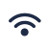•• Garage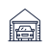•••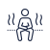•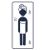•• Slopes access :
• ski-in (-50 m)
• Rooms :
• 2 rooms + cabin(s)
• Total surface (sq.m) :
• 41 sq.m
• Slopes access :
• ski-in (-50 m)
• Rooms :
• Studio
• Studio + cabin(s)
• Total surface (sq.m) :
• 30 sq.m
• Garage•••• Slopes access :
• ski-in (-50 m)
• Rooms :
• 2 rooms
• 2 rooms + cabin(s)
• Total surface (sq.m) :
• 42 sq.m
••••• Slopes access :
• 50 -300m to the slopes
• Rooms :
• 2 rooms
• Total surface (sq.m) :
• 45 sq.m
••••• Slopes access :
• ski-in (-50 m)
• Rooms :
• 3 rooms
• Total surface (sq.m) :
• 42 sq.m
••••• Slopes access :
• 50 -300m to the slopes
• Rooms :
• 2 rooms
• 2 rooms + cabin(s)
• Total surface (sq.m) :
• 27 sq.m
•••• Slopes access :
• ski-in (-50 m)
• Rooms :
• 4 rooms
• Total surface (sq.m) :
• 66 sq.m
••••• Slopes access :
• ski-in (-50 m)
• Rooms :
• 2 rooms + cabin(s)
• Total surface (sq.m) :
• 40 sq.m
••••• Slopes access :
• ski-in (-50 m)
• Rooms :
• 2 rooms
• 2 rooms + cabin(s)
• Total surface (sq.m) :
• 65 sq.m
•••••• Slopes access :
• 50 -300m to the slopes
• Rooms :
• 4 rooms
• 4 rooms + cabin(s)
• Total surface (sq.m) :
• 120 sq.m
••••